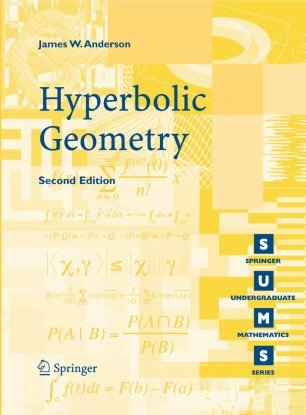# Hyperbolic GeometryTextbook

Part of the Springer Undergraduate Mathematics Series book series (SUMS)

1. Front Matter
Pages i-xi
2. Pages 1-21
3. Pages 23-72
4. Pages 73-115
5. Pages 117-144
6. Pages 145-187
7. Pages 189-215
8. Back Matter
Pages 217-276

### Introduction

The geometry of the hyperbolic plane has been an active and fascinating field of mathematical inquiry for most of the past two centuries. This book provides a self-contained introduction to the subject, suitable for third or fourth year undergraduates. The basic approach taken is to define hyperbolic lines and develop a natural group of transformations preserving hyperbolic lines, and then study hyperbolic geometry as those quantities invariant under this group of transformations.

Topics covered include the upper half-plane model of the hyperbolic plane, Möbius transformations, the general Möbius group, and their subgroups preserving the upper half-plane, hyperbolic arc-length and distance as quantities invariant under these subgroups, the Poincaré disc model, convex subsets of the hyperbolic plane, hyperbolic area, the Gauss-Bonnet formula and its applications.

This updated second edition also features:

an expanded discussion of planar models of the hyperbolic plane arising from complex analysis;

the hyperboloid model of the hyperbolic plane;

brief discussion of generalizations to higher dimensions;

many new exercises.

The style and level of the book, which assumes few mathematical prerequisites, make it an ideal introduction to this subject and provides the reader with a firm grasp of the concepts and techniques of this beautiful part of the mathematical landscape.

### Keywords

Area Geometry Hyperbolic geometry Hyperbolic plane Hyperbolicity Polygon calculus mathematics